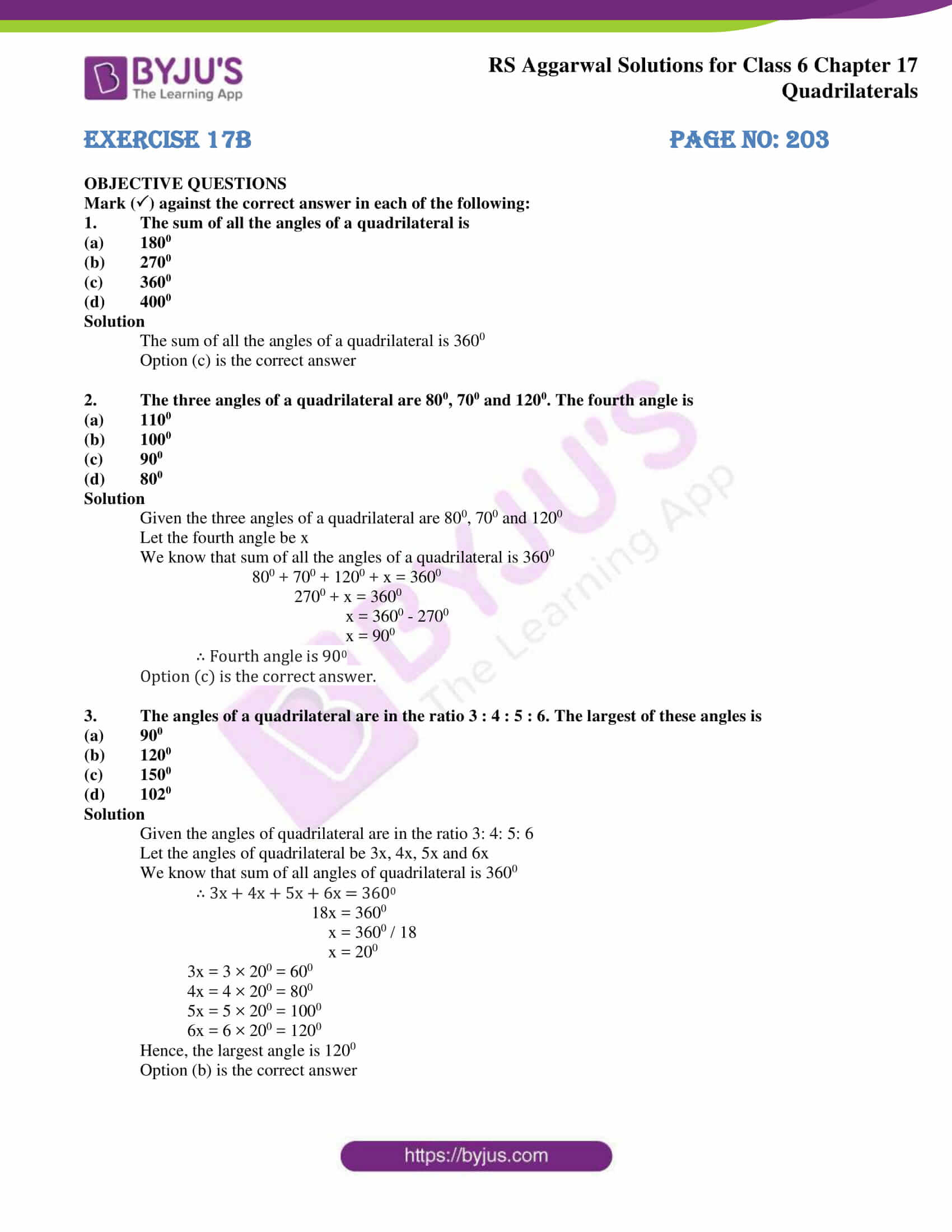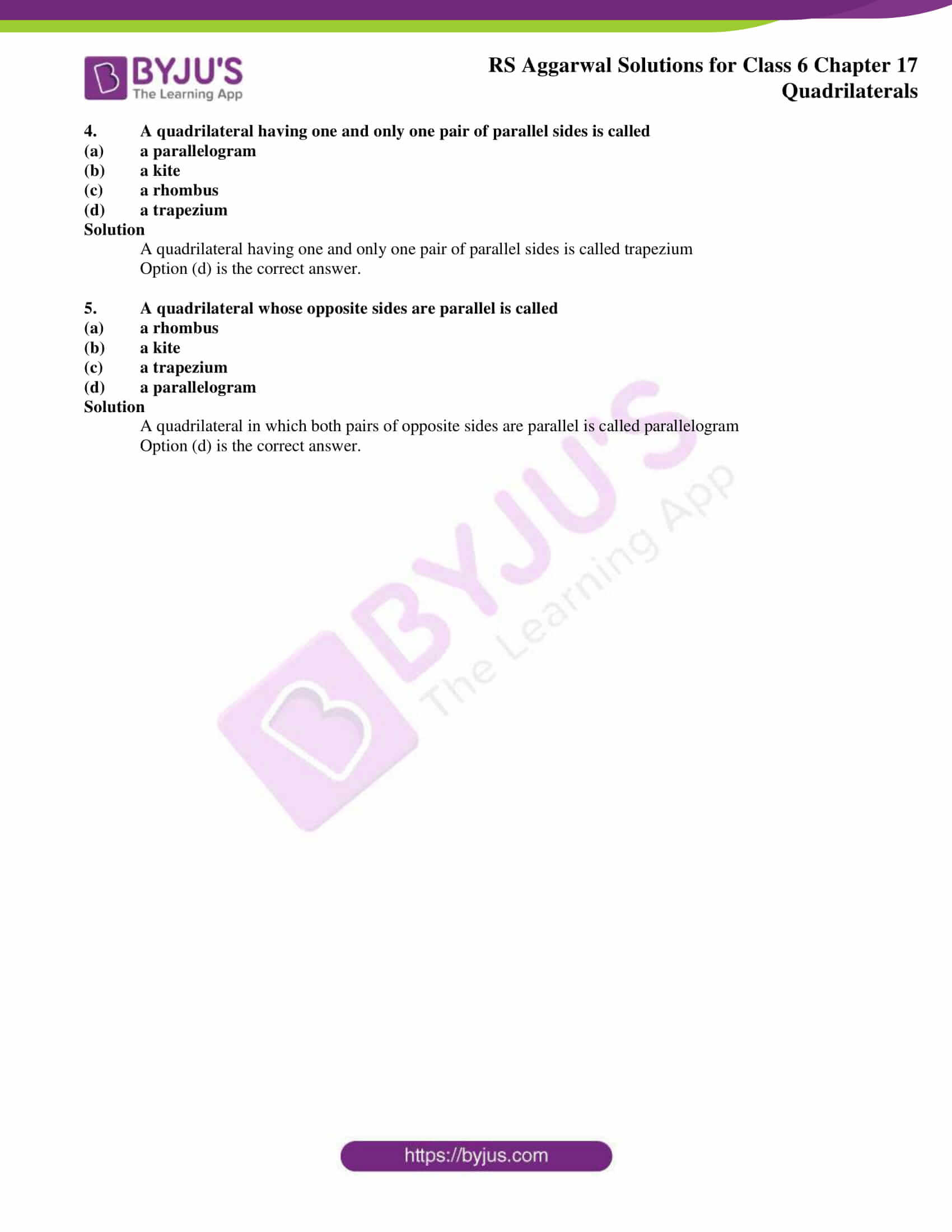# RS Aggarwal Solutions for Class 6 Chapter 17 Quadrilaterals Exercise 17B

The students must have good knowledge about the concepts which are covered in Class 6 as they are significant in scoring good marks in higher grades. RS Aggarwal Solutions are designed by expert faculty based on the grasping capacity of students. The students can solve exercise wise problems with the help of solutions to understand the other methods of solving the same problem. The faculty at BYJU’S have prepared the RS Aggarwal Solutions for Class 6 Chapter 17 Quadrilaterals Exercise 17B which is available here in PDF format.### Access answers to Maths RS Aggarwal Solutions for Class 6 Chapter 17 Quadrilaterals Exercise 17B

OBJECTIVE QUESTIONS

Mark () against the correct answer in each of the following:

1. The sum of all the angles of a quadrilateral is

(a) 1800

(b) 2700

(c) 3600

(d) 4000

Solution

The sum of all the angles of a quadrilateral is 3600

Option (c) is the correct answer

2. The three angles of a quadrilateral are 800, 700 and 1200. The fourth angle is

(a) 1100

(b) 1000

(c) 900

(d) 800

Solution

Given the three angles of a quadrilateral are 800, 700 and 1200

Let the fourth angle be x

We know that sum of all the angles of a quadrilateral is 3600

800 + 700 + 1200 + x = 3600

2700 + x = 3600

x = 3600 – 2700

x = 900

∴ Fourth angle is 900

Option (c) is the correct answer.

3. The angles of a quadrilateral are in the ratio 3 : 4 : 5 : 6. The largest of these angles is

(a) 900

(b) 1200

(c) 1500

(d) 1020

Solution

Given the angles of quadrilateral are in the ratio 3: 4: 5: 6

Let the angles of quadrilateral be 3x, 4x, 5x and 6x

We know that sum of all angles of quadrilateral is 3600

∴ 3x + 4x + 5x + 6x = 3600

18x = 3600

x = 3600 / 18

x = 200

3x = 3 × 200 = 600

4x = 4 × 200 = 800

5x = 5 × 200 = 1000

6x = 6 × 200 = 1200

Hence, the largest angle is 1200

Option (b) is the correct answer

4. A quadrilateral having one and only one pair of parallel sides is called

(a) a parallelogram

(b) a kite

(c) a rhombus

(d) a trapezium

Solution

A quadrilateral having one and only one pair of parallel sides is called trapezium

Option (d) is the correct answer.

5. A quadrilateral whose opposite sides are parallel is called

(a) a rhombus

(b) a kite

(c) a trapezium

(d) a parallelogram

Solution

A quadrilateral in which both pairs of opposite sides are parallel is called parallelogram

Option (d) is the correct answer.

### Access other exercises of RS Aggarwal Solutions for Class 6 Chapter 17 Quadrilaterals

Exercise 17A Solutions